﻿ 重载货车轮对弹性振动模态阶数对轮轨动态作用的影响

重载货车轮对弹性振动模态阶数对轮轨动态作用的影响Influence of Wheelset Elastic Vibration Mode Order on Wheel-Rail Dynamic Interaction of Heavy-Haul Freight Wagon

Abstract: To study the influence of elastic vibration mode order of wheelset on wheel-rail dynamic interaction, a 30 t axle-load wagon was taken as the research object. Firstly, the elastic models of wheelset with different vibrating modes were established by using the finite element method, which were introduced into the dynamic software to constitute the rigid-elastic coupling dynamic model of freight wagon. The time domain, frequency domain and statistical maximum analysis methods were applied. It was found that, the first-order lateral bending mode of wheelset and umbrella-type vibration mode of wheels could ease the wheel/rail lateral and vertical forces. Meanwhile, the first-order horizontal and vertical bending modes would produce larger longitudinal creep forces. Finally, the influence of different modes on the dynamics at different speeds was analyzed. As the first-order torsion, lateral bending and second-order vertical bending modes were excited, the change of velocity had great influence on the creep forces of wheelset. Comparing between the wheel-rail lateral and vertical forces, the speed change had the greater influence on the wheel-rail lateral forces when the first-order and second-order vertical bending vibration modes dominated in the wheelset.

1. 引言

2. 柔性轮对的动力学建模方法

2.1. 柔性体的运动学描述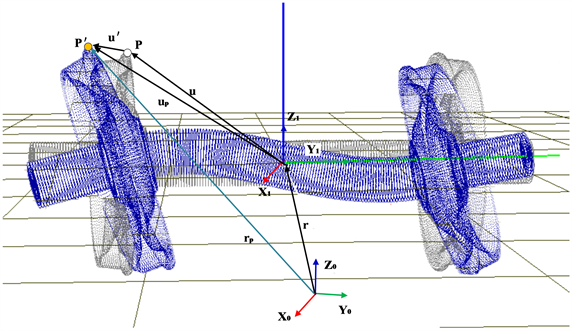Figure 1. Description of elastomer space

${r}_{k}={r}_{1}+{A}_{01}\left(u+{u}^{\prime }\right)$ (1)

${u}^{\prime }={H}_{P}{q}_{\left(t\right)}$ (2)

2.2. 轮对柔性体的有限元建模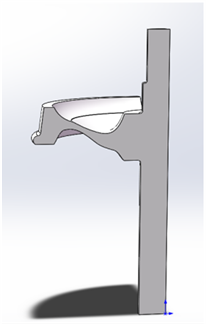Figure 2. Wheelset structure drawing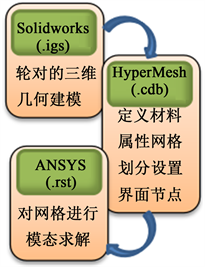Figure 3. Modeling process of flexible wheelset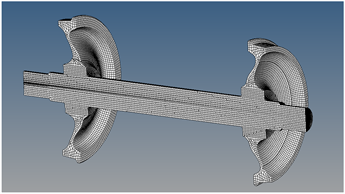Figure 4. Finite element model of wheelset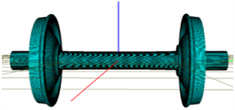(a) 一阶扭转(f = 98.84)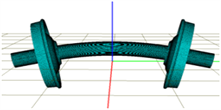(b) 一阶垂弯(f = 172.21)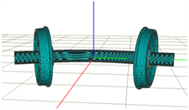(c) 一阶横弯(f = 172.21)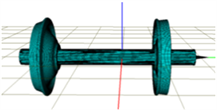(d) 二阶伞形(f = 246.94)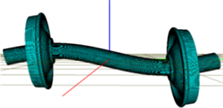(e) 二阶垂弯(f = 274.75)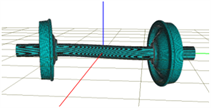(f) 二阶横弯(f = 274.75)

Figure 5. Alone modal models and corresponding frequencies

2.3. 刚柔耦合动力学模型的集成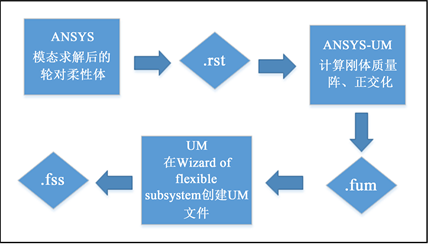Figure 6. UM wheelset handling processFigure 7. Heavy-haul freight wagon topological relationship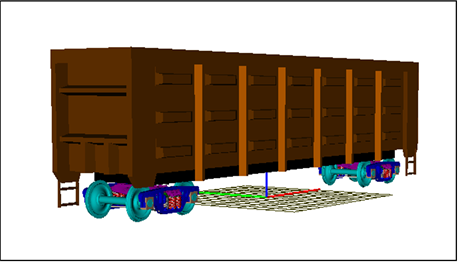Figure 8. Dynamics model

2.4. 轨道不平顺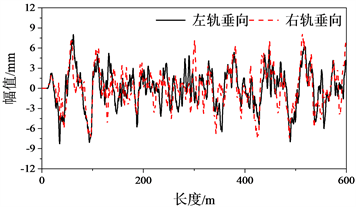(a) 垂向不平顺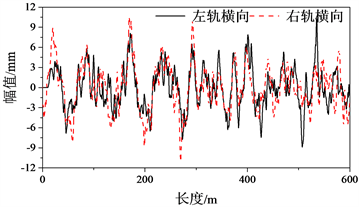(b) 横向不平顺

Figure 9. Track irregularity

3. 模态阶数间的车辆动力学的影响分析

3.1. 模态阶数模型间的动力学响应对比分析(a) 横向振型时域响应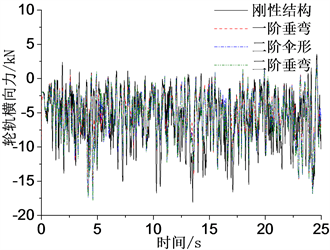(b) 垂向振型时域响应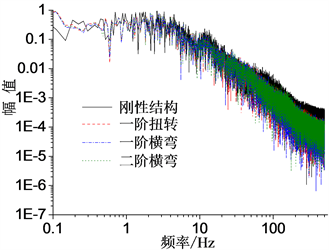(c) 横向振型频谱特性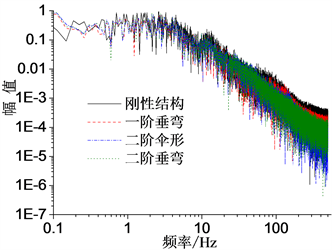(d) 垂向振型频谱特性

Figure 10. Time-domain response and spectrum characteristics of wheel-rail lateral forces in different modes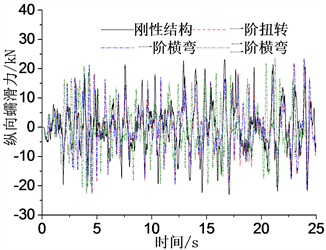(a) 横向振型时域响应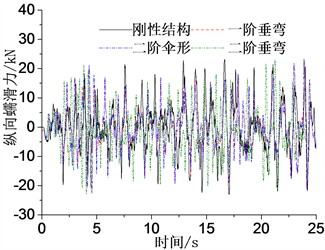(b) 垂向振型时域响应(c) 横向振型频谱特性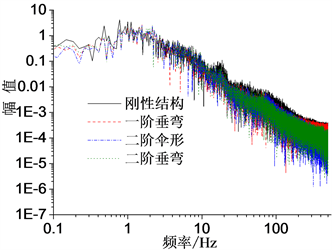(d) 垂向振型频谱特性

Figure 11. Time-domain response and spectrum characteristics of wheel-rail longitudinal creep forces in different modes

3.2. 模态阶数模型的动力学响应统计最大值对比分析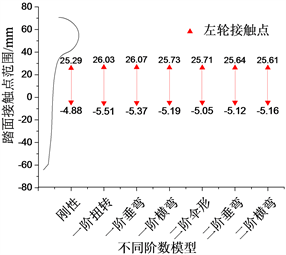(a) 左轮踏面接触点位置对比图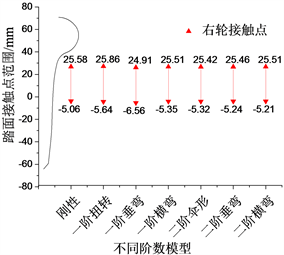(b) 右轮踏面接触点位置对比图

Figure 12. Comparison diagram of the position of contact point of one wheelset tread with different mode models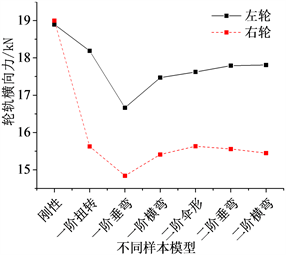(a) 轮轨横向力对比图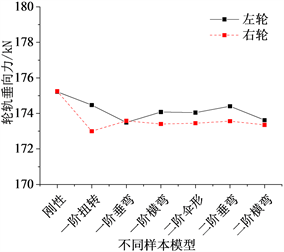(b) 轮轨垂向力对比图

Figure 13. Comparison diagram of wheel-rail force of one wheelset tread with different mode models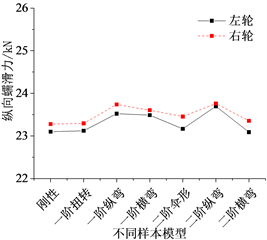(a) 纵向蠕滑力对比图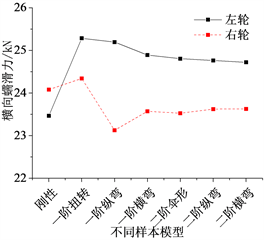(b) 横向蠕滑力对比图

Figure 14. Comparison diagram of creep force of one wheelset tread with different mode models

4. 不同速度对弹性振动模态阶数影响分析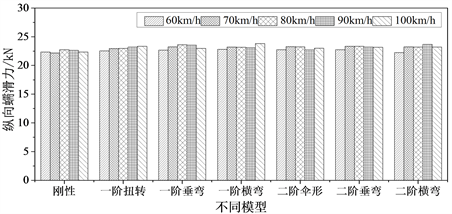(a) 纵向蠕滑力统计最大值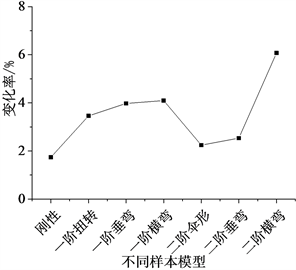(b) 纵向蠕滑力变化率

Figure 15. The statistical maximum value and change rate of longitudinal creep force of different velocities all the modes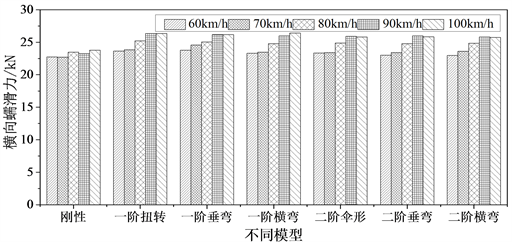(a) 横向蠕滑力统计最大值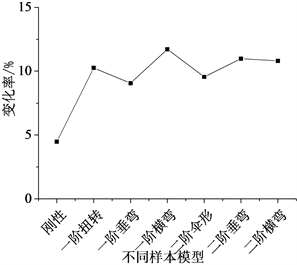(b) 横向蠕滑力变化率

Figure 16. The statistical maximum value and change rate of lateral creep force of different velocities all the modes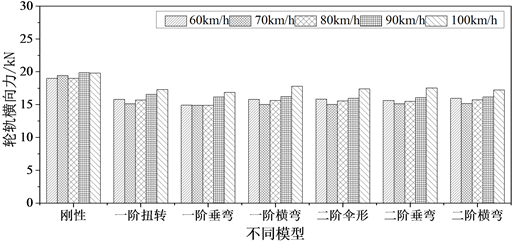(a) 横向蠕滑力统计最大值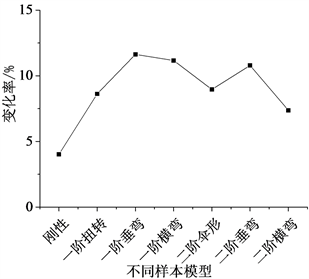(b) 横向蠕滑力变化率

Figure 17. The statistical maximum value and change rate of Wheel-rail lateral force of different velocities all the modes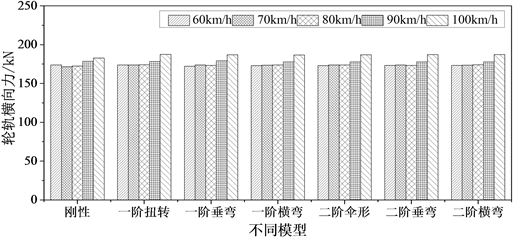(a) 轮轨垂向力统计最大值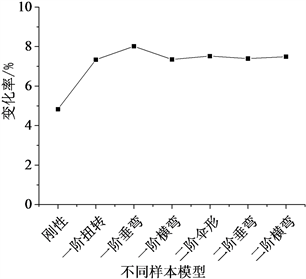(b) 轮轨垂向力变化率

Figure 18. The statistical maximum value and change rate of Wheel-rail vertical force of different velocities all the modes

5. 结论

1) 通过对弹性振动模态阶数模型间的时域、频域对比可知，轮对的弹性结构能缓和轮轨横、垂向力，尤其体现在一阶横弯模态和二阶伞形；与此同时一阶横、垂弯模态会产生较大的轮轨蠕滑力，可能是造成轮对的局部磨耗和车轮失圆的一个重要因素。

2) 分析统计最大值可知，轮对的弹性振动一阶模态能够使轮轨作用力有较大的改变。尤其是一阶垂弯，较大程度上缓和了轮轨刚性作用，所以在建模时，只把轮对考虑成刚性结构会过高的评估轮轨动态作用；与此同时一、二阶垂弯和一阶扭转模态会造成较大蠕滑力，可能是导致轮轨磨损的重要原因。

3) 通过分析不同速度对弹性振动模态阶数的影响可知，对比不同模态阶数模型的轮轨蠕滑力，速度的改变对一阶扭转、横弯以及二阶垂弯模态的影响最大；对于轮轨横、垂向作用力，当轮对发生以一阶、二阶垂弯为主的振动时，速度对轮轨横向力的影响较为明显。说明速度的改变对弹性振动模态阶数的影响不容忽视。

 韩鹏, 张卫华. 轮对结构弯曲及型面磨耗对高速列车振动性能的影响[J]. 振动与冲击, 2015, 34(5): 207-212.

 Andersson, C. and Abrahamsson, T. (2000) Simulation of Interaction between a Train in General Motion and a Track. Department of Solid Mechanics, Chalmers University of Technology, Göteborg.

 Andersson, C., Oscarsson, J. and Nielsen, J. (2000) Dynamic Interaction between a Flexible Vehicle and a State-Dependent Railway Track. Department of Solid Mechanics, Chalmers University of Technology, Göteborg.

 Brommundt, E. (1997) A Simple Mechanism for the Polygonalization of Railway Wheels by Wear. Mechanics Research Communications, 24, 435-442.
https://doi.org/10.1016/S0093-6413(97)00047-5

 李伟, 李言义, 张雄飞, 等. 地铁车辆车轮多边形的机理分析[J]. 机械工程学报, 2013, 49(18): 17-22.

 杨亮亮, 罗世辉, 傅茂海, 等. 车轮状态变化对重载货车轮作用力影响[J]. 振动与冲击, 2014, 33(3): 110-116.

 方翁武, 刘韦, 罗世辉, 等. 轮对多边形化对车辆动力学性能的影响[J]. 机车电传动, 2013(4): 59-62.

 罗仁, 曾京, 邬平波, 戴焕云. 高速列车车轮不圆顺磨耗仿真及分析[J]. 铁道学报, 2011, 32(5): 30-36.

 郭训, 郑树彬, 柴晓冬, 李立明. 基于柔性轮对的轨道车辆动力学仿真分析[J]. 测控技术, 2018, 37(8): 150-153.

 李国芳, 姚永明, 丁旺才. 基于UM的车辆-轨道耦合动力学建模及仿真分析[J]. 兰州交通大学学报, 2016, 35(1): 142-146.

 翟婉明. 车辆-轨道耦合动力学[M]. 第4版. 北京: 科学出版社, 2015.

 刘鹏飞, 翟婉明, 王开云, 田国英. 考虑轨道弹性时机车轮对的纵向振动研究[J]. 工程力学, 2013, 30(12): 256-262.

 王勇, 曾京, 张卫华. 铁路货车非线性稳定性[J]. 交通运输工程学报, 2002, 2(2): 36-40.

 徐磊, 陈宪麦, 李晓健, 孟宪洪. 朔黄重载铁路轨道不平顺谱分析[J]. 中南大学学报(自然科学版), 2013, 44(12): 5147-5153.

Top